Subject:
Numbers and Operations
Material Type:
Lesson Plan
Level:
Middle School
7
Provider:
Pearson
Tags:
• Multiplication
• Negative Numbers
Language:
English
Media Formats:
Text/HTML

# Model Integers Multiplication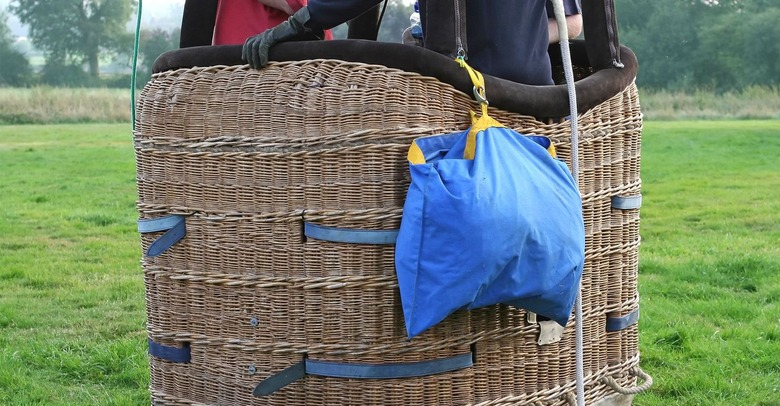## Overview

Students use number lines to represent products of a negative integer and a positive integer, and they use patterns to understand products of two negative integers. Students write rules for products of integers.

# Key Concepts

The product of a negative integer and a positive integer can be interpreted as repeated addition. For example, 4 • (–2) = (–2) + (–2) + (–2) + (–2). On a number line, this can be represented as four arrows of length 2 in a row, starting at 0 and pointing in the negative direction. The last arrow ends at –8, indicating that 4 • (–2) = –8. In general, the product of a negative integer and a positive integer is negative.

The product of two negative integers is hard to interpret or visualize. In this lesson, we use patterns to help students see why a negative integer multiplied by a negative integer equals a positive integer. For example, students can compute the products in the pattern below.

4 • (–3) = –12

3 • (–3) = –9

2 • (–3) = –6

1 • (–3) = –3

0 • (–3) = 0

They can observe that, as the first factor decreases by 1, the product increases by 3. They can continue this pattern to find these products.

–1 • (–3) = 3

–2 • (–3) = 6

–3 • (–3) = 9

In the next lesson, we will prove that the rules for multiplying positive and negative integers extend to all rational numbers, including fractions and decimals.

# Goals and Learning Objectives

• Represent multiplication of a negative integer and a positive integer on a number line.
• Use patterns to understand products of two negative integers.
• Write rules for multiplying integers.

# Lesson Guide

Ask a student to remind the class about the balloon model and how the balloon moves when heat or weight is added or removed. Then give partners a minute to read the Opening and represent the situation. Check to be sure everyone has a correct number line and equations before moving to Work Time. Students should see that because (−2) + (−2) + (−2) + (−2) is the sum of four –2s, it can be written as the multiplication 4 ⋅ (−2).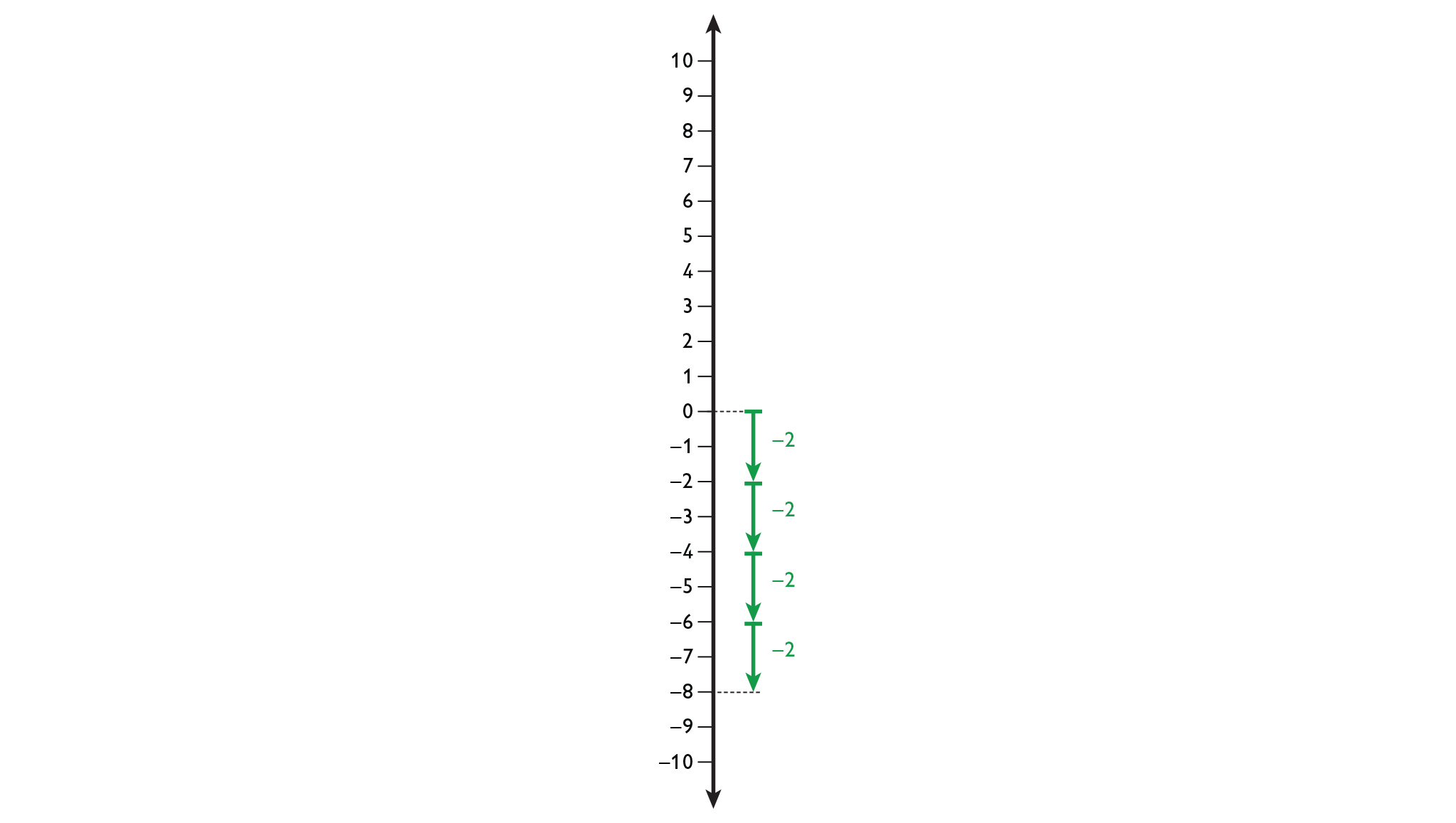•  (−2) + (−2) + (−2) + (−2) = −8

•  4 ⋅ (−2) = −8

# Hot Air Balloon

Think back to the Hot Air Balloon simulation you worked with earlier in this unit. Then consider the following situation:

Suppose the balloon starts at 0, and you add 2 units of weight to the balloon every minute for 4 minutes. Where will the balloon be after 4 minutes?

Represent this situation in each of the following ways.

• On the number line
• As a multiplication equation

## Hint:

• Remember that weight represents a negative number.
• How many units of –2 are added together?
• How can you represent repeated addition as multiplication?
• Each time 2 units of weight are added to the hot air balloon, the balloon's altitude decreases by 2 units.

# Math Mission

Discuss the Math Mission. Students will use number lines and patterns to multiply integers and write rules for multiplying integers.

## Opening

Use number lines and patterns to multiply integers and write rules for multiplying integers.

# Lesson Guide

Students should work in pairs on the problems.

Look for different rules that students wrote to present during Ways of Thinking. If anyone wrote an incorrect rule, be sure it is presented.

ELL: Encourage ELLs to use their English speaking skills in class. At the same time, allow students to use their language of origin to clarify concepts during partner work. New ELLs may benefit from partners who share the same primary language.

# Mathematics

Students who think of the product m · n as the sum of m number of ns may have difficulty representing –3 · 3. Remind them that multiplication is commutative, so the order of the factors does not matter. Therefore, –3 · 3 is the same as 3 · (–3), or the sum of three –3s.

# Mathematical Practices

Mathematical Practice 7: Look For and Make Use of Structure

Writing the product rule for a specific case, then generalizing it, requires finding and extending patterns.

# Interventions

Student has difficulty representing –3 · 3 on a number line.

• Does the order in which the factors in a multiplication are written matter?
• What is another way of writing −3 ⋅ 3?
• How can you write this expression as a repeated addition?
• How do you represent the addition on the number line?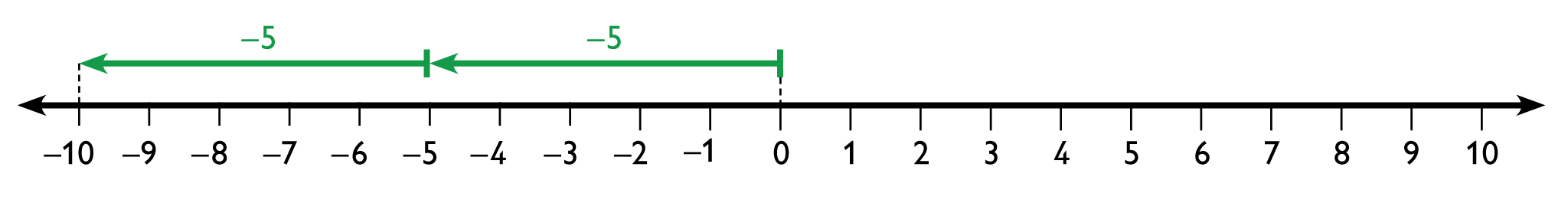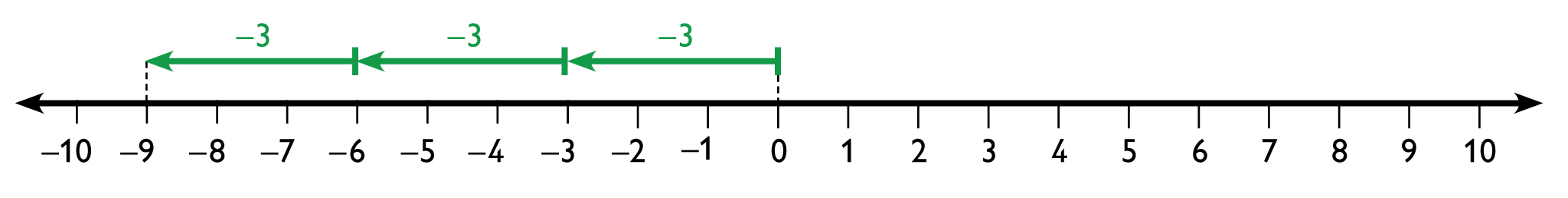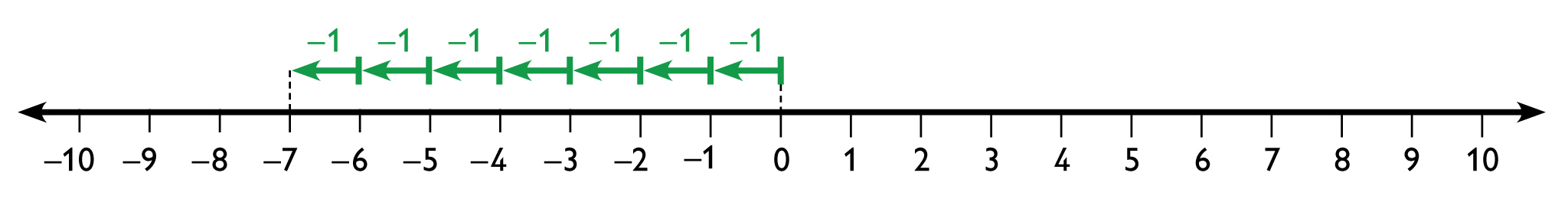• 2 ⋅ (−5) = −10
• −3 ⋅ 3 = −9
• 7 ⋅ (−1) = −7
• Answers will vary. Possible answer: The product of a positive integer and a negative integer is always negative.

# A Positive Times a Negative

• Represent each multiplication problem on a number line. Write the product as an equation.
• 2 ⋅ (−5)
• −3 ⋅ 3
• 7 ⋅ (−1)
• Write a rule about the sign of the product of a positive integer and a negative integer.

## Hint:

• Regardless of the order of the factors, the positive integer will always indicate the number of times that the negative integer should be repeated.
• How does the product compare to the product you would get if you ignored the negative sign?

# Lesson Guide

Students should continue to work in pairs.

If students struggle with completing the table, remind them that they just wrote a rule they can use to find the first several products. They might also find it useful to represent some of the expressions on a number line.

Questions to pose while students are completing the table:

• What pattern do you see in the equations so far?
• How is the product changing each time the first factor decreases by 1?
• If this pattern continues, what will the next product be? What about the product after that?
• If you multiply any number times 0, what is the product?

–3 · 3 is the same as 3 · (–3), or the sum of three –3s.

# Mathematical Practices

Mathematical Practice 8: Look for and Express Regularity in Repeated Reasoning

To find the rules for multiplying integers, students look for repeated calculations and then generalize them. As students work through the problems, they may notice that the product of a negative integer and a positive integer is the same as the product of the numbers without the signs, but it is negative. They can use this general rule to quickly find products of two integers with different signs.

# Interventions

Student does not recognize the pattern in the products in the table.

• What is the same about all the equations?
• What happens to the first factor as we go from one equation to the next?
• What happens to the product as we go from one equation to the next?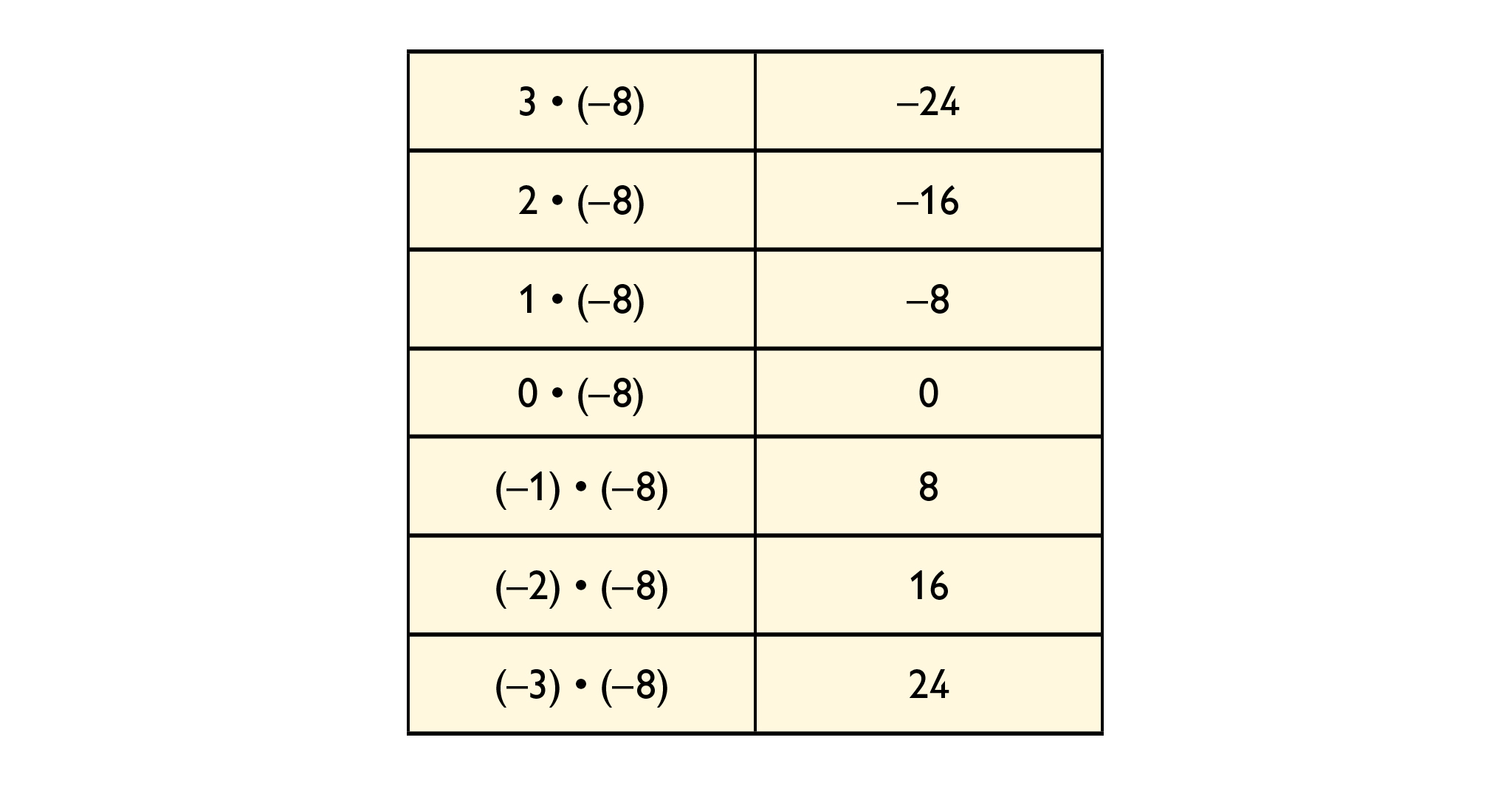• The product of two numbers with the same sign is positive.
• The product of two numbers with different signs is negative.

# Challenge Problem

• $-{2}^{4}=-16$
• ${\left(-2\right)}^{3}=-8$
• −(−7)2 = −49
• ${5}^{3}=125$
• For odd values of m, $-{p}^{m}={\left(-p\right)}^{m}$.
• For odd values of m, $-{n}^{m}={\left(-n\right)}^{m}$.

# Multiplying Integers

Identify and use patterns in the table to help you evaluate the expressions.

• The product of two numbers with the same sign is ____.
• The product of two numbers with different signs is _____.

# Challenge Problem

Recall that the notation ${a}^{m}$ means to multiply m instances of a.
Example: ${2}^{4}$ = 2 ⋅ 2 ⋅ 2 ⋅ 2

The expression ${\left(-a\right)}^{m}$ means to multiply m instances of the opposite of a (that is, m instances of –a ). The notation ${-a}^{m}$ means to multiply m instances of a and then find the opposite of the result.

• Find the value of each expression.
• $-{2}^{4}$ =
• ${\left(-2\right)}^{3}$ =
• $-{\left(-7\right)}^{2}$ =
• ${5}^{3}$ =
• Suppose p is a positive integer. For what values of m does $-{p}^{m}={\left(-p\right)}^{m}$?
• Suppose n is a negative integer. For what values of m does $-{n}^{m}={\left(-n\right)}^{m}$?

## Hint:

• What happens to the products as the first factor decreases by 1?
• How does the product compare to the product you would get if the factor was positive?

# Lesson Guide

Students should state their rule for multiplying a positive integer and a negative integer and then give an example to demonstrate their rule.

Have different students present the table. The student should complete the first few equations, explain the pattern, and then explain how to extend the pattern to complete the rest of the equations.

Ask a student to present the solutions to the Challenge Problem. Have the student show that their conclusions about m are correct by demonstrating with examples.

SWD: Students with disabilities may struggle to follow the discussion and concurrently take notes during the Ways of Thinking portion of the lesson. Create scaffolded notes/questions based on the responses for some students. Use the notes as reference and support during the Ways of Thinking portion of the lesson.

# Ways of Thinking: Make Connections

## Hint:

• How did you make your number line?
• Can you describe a pattern that you identified in the table?
• How did you use the pattern to evaluate the expression?

# Lesson Guide

Students should work alone. Tell them that they will need to combine the rules and methods developed earlier in the lesson to solve these problems.

Students may need to be reminded that the commutative and associative properties apply to multiplication as well as addition.

• 2 ⋅ (−2) = −4
• −4 ⋅ (−1) = 4
• 2 ⋅ (−2) ⋅ (−4) ⋅ (−1) = −16
• −1 ⋅ (−6) ⋅ (−2) ⋅ (−3) ⋅ 2 = 72
• Answers will vary. Possible answer: If there is an even number of negative numbers, all of the negative numbers can be paired off so that their products will be positive, and therefore the whole product will be positive. If there is an odd number of negative numbers, the negative numbers can be paired off so that their products will be positive, with one negative number left. The result will be a product of positive numbers with one negative number.

# Multiply Integers

• Find the product of each equation.
• 2 ⋅ (−2) =
• −4 ⋅ (−1) =
• 2 ⋅ (−2) ⋅ (−4) ⋅ (−1) =
• −1 ⋅ (−6) ⋅ (−2) ⋅ (−3) ⋅ 2 =
• How do you know whether the product of several integers will be positive or negative? Justify your answer.

# Lesson Guide

Have students discuss the summary with a partner before turning to a whole class discussion. Use this opportunity to correct or clarify misconceptions. It may be helpful to have students write numerical examples or draw a number line for each of the statements.

ELL: Write the key points on a poster so that students can refer back to them throughout the module. Provide supplementary materials, such as graphic organizers, to illustrate new concepts and vocabulary necessary for mathematical learning.

# Mathematics

The product of a positive integer and a negative integer can be modeled on a number line. Start at 0 and draw arrows end-to-end to show the negative integer a number of times equal to the positive integer. So, for example, to show 3 · (–4), start at 0 and draw 3 arrows of length 4 units pointing in the negative direction. The product of a negative integer and a positive integer is negative.

To show the product of two negative integers, we can write a series of products, starting with a positive integer times the second factor and decreasing the first factor by 1 each time. We can look for a pattern in the products and extend it until we get to the product of two negative integers. For example:

3 ⋅ (−6) = −182 ⋅ (−6) = −121 ⋅ (−6) = −60 ⋅ (−6) = 0 − 1 ⋅ (−6) = 6 − 2 ⋅ (−6)
= 12 − 3 ⋅ (−6) = 18 − 4 ⋅ (−6) = 24

SWD: Reflecting on learning can be challenging for some students. Support students who may get lost in the meaning of this task by providing specific questions to which they can respond.

# Summary of the Math: Rules for Multiplying Integers

• A positive number multiplied by a positive number results in a positive number.
• A negative number multiplied by a negative number results in a positive number.
• A positive number multiplied by a negative number results in a negative number.
• A negative number multiplied by a positive number results in a negative number.
• Multiplying by a negative number is the same as taking the opposite.
ab is the opposite of −(ab), or −1(ab).
• When multiplying a negative number by a negative number, the opposite of an opposite is the number itself.
a ⋅ −b is the opposite of −1(ab), and −1(ab) is the opposite of ab. Since a negative number times a negative number results in a positive number, we can conclude that the opposite of an opposite is the number itself, or −a ⋅ −b = ab.

## Hint:

Can you:

• Describe how to represent the product of a positive integer and a negative integer on a number line?
• Explain how you can use patterns to show that the product of two negative integers is positive?
• Explain how you know what the sign of the product of any two integers will be?

# Lesson Guide

Have each student write a brief reflection before the end of the class. Review the reflections to find out what students have learned about multiplying integers.

Support students as they reflect on their learning by providing models from earlier lessons that include the elements of a complete reflection.

Create a “reflection checklist” to help students self-assess the quality of their reflections. This will help struggling writers to self-monitor their work.

# Reflection

Write a reflection about the ideas discussed in class today. Use the sentence starter below if you find it to be helpful.

Something new I learned today is ...